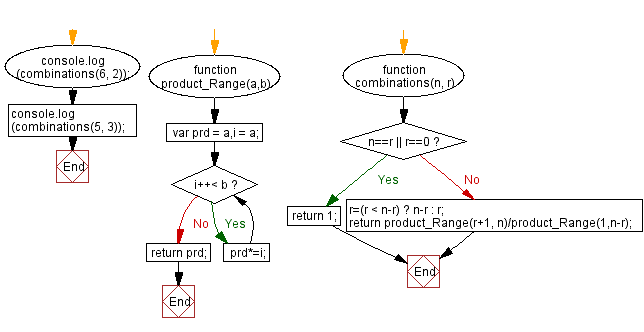# JavaScript: Calculate the combination of n and r

## JavaScript Math: Exercise-42 with Solution

Write a JavaScript function to calculate the combination of n and r.
The formula is : n!/(r!*(n - r)!).

Test Data :
console.log(combinations(6, 2));
15
console.log(combinations(5, 3));
10

Sample Solution:-

HTML Code:

``````<!DOCTYPE html>
<html>
<meta charset="utf-8">
<title>JavaScript function to calculate the combination of n and r. The formula is : n!/(r!*(n - r)!)</title>
<body>

</body>
</html>
```
```

JavaScript Code:

``````function product_Range(a,b) {
var prd = a,i = a;

while (i++< b) {
prd*=i;
}
return prd;
}

function combinations(n, r)
{
if (n==r || r==0)
{
return 1;
}
else
{
r=(r < n-r) ? n-r : r;
return product_Range(r+1, n)/product_Range(1,n-r);
}
}

console.log(combinations(6, 2));
console.log(combinations(5, 3));
```
```

Sample Output:

```15
10
```

Flowchart:Live Demo:

See the Pen javascript-math-exercise-42 by w3resource (@w3resource) on CodePen.

Improve this sample solution and post your code through Disqus

What is the difficulty level of this exercise?

Test your Programming skills with w3resource's quiz.

﻿